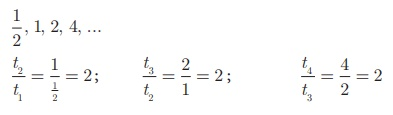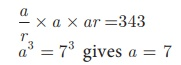Home | | Maths 10th Std | Geometric Progression

# Geometric Progression

A Geometric Progression is a sequence in which each term is obtained by multiplying a fixed non-zero number to the preceding term except the first term. The fixed number is called common ratio. The common ratio is usually denoted by r.

Geometric Progression

In the diagram given in Fig.2.13, ΔDEF is formed by joining the mid points of the sides AB, BC and CA of ΔABC. Then the size of the triangle ΔDEF is exactly one-fourth of the size of ΔABC. Similarly ΔGHI is also one-fourth of ΔFDE and so on. In general, the successive areas are one-fourth of the previous areas.

The area of these triangles are D ABC,In this case, we see that beginning with ΔABC, we see that the successive triangles  are formed whose  areas are precisely one-fourth the area of the previous triangle. So, each term is obtained by multiplying 1/4 to the previous term.As another case, let us consider that a viral disease is spreading in a way such that at any stage two new persons get affected from an affected person. At first stage, one person is affected, at second stage two persons are affected and is spreading to four persons and so on. Then, number of persons affected at each stage are 1, 2, 4, 8, ... where except the first term, each term is precisely twice the previous term.

From the above examples, it is clear that each term is got by multiplying a fixed number to the preceding number.

This idea leads us to the concept of Geometric Progression.

## Definition

A Geometric Progression is a sequence in which each term is obtained by multiplying a fixed non-zero number to the preceding term except the first term. The fixed number is called common ratio. The common ratio is usually denoted by r.

## 1. General form of Geometric Progression

Let a and r ≠ 0 be real numbers. Then the numbers of the form a , ar , ar2 , ... arn-1... is called a Geometric Progression. The number ‘a’ is called the first term and number ‘r’ is called the common ratio.

We note that beginning with first term a, each term is obtained by multiplied with the common ratio ‘r’ to give ar , ar 2 ,ar 3,...

## 2. General term of Geometric Progression

We try to find a formula for nth term or general term of Geometric Progression (G.P.) whose terms are in the common ratio.

a , ar , ar2 ,..., arn-1, ... where a is the first term and ‘r’ is the common ratio. Let tn  be the nth term of the G.P.

Then

t1 = a = a × r0   = a ×r1−1

t2 = t1 × r = a × r = a × r2−1

t3 = t2 × r = ar × r = ar2 = ar3−1

:            :

tn = tn −1 × r = arn −2 × r = arn −2+1 = ar n−1

Thus, the general term or nth  term of a G.P. is tn = arn-1

Note

If we consider the ratio of successive terms of the G.P. then we haveThus, the ratio between any two consecutive terms of the Geometric Progression is always constant and that constant is the common ratio of the given Progression.

### Example 2.40

Which of the following sequences form a Geometric Progression?

(i) 7, 14, 21, 28, …

(ii) 1/ 2 , 1, 2, 4, ...

(ii) 5, 25, 50, 75, …

### Solution

To check if a given sequence form a G.P. we have to see if the ratio between successive terms are equal.

(i) 7, 14, 21, 28, …Since the ratios between successive terms are not equal, the sequence 7, 14, 21, 28, … is not a Geometric Progression.

(ii)Here the ratios between successive terms are equal. Therefore the sequence 1/2 , 1, 2, 4, ... is a Geometric Progression with common ratio r= 2.

(iii) 5, 25, 50, 75,...Since the ratios between successive terms are not equal , the sequence 5, 25, 50, 75,... is not a Geometric Progression.

### Example 2.41

Find the geometric progression whose first term and common ratios are given by

(i) a = −7 , r = 6

(ii) a = 256 , r = 0.5

### Solution

(i) The general form of Geometric progression is a, ar, ar2 ,.. .

a = −7 , ar = −7 × 6 = −42 , ar 2  = −7 × 62  = −252

(ii) The general form of Geometric progression is a, ar, ar2 ,...

a = 256 , ar = 256 × 0.5 = 128 , ar 2  = 256 ×(0.5)2  = 64

Therefore the required Geometric progression is 256,128, 64,....

### Example 2.42

Find the 8th term of the G.P. 9, 3, 1,…

### Solution

To find the 8th term we have to use the nth term formula tn  = arn−1

First term a = 9 , common ratio rTherefore the 8th term of the G.P. is 1/243

### Example 2.43

In a Geometric progression, the 4th term is 8/9 and the 7th term is 64/243. Find the Geometric Progression.

### SolutionSubstituting the value of r in (1), we get a × [2/3]3 = 8/9 a = 3

Therefore the Geometric Progression is a, ar, ar2 , … That is, 3, 2, 4/3,…..

Note

·           When the product of three consecutive terms of a G.P. are given, we can take the three terms as a/r , a, ar.

·           When the products of four consecutive terms are given for a G.P. then we can take the four terms as a/r3 , a/r , ar, ar 3 .

·           When each term of a Geometric Progression is multiplied or divided by a non– zero constant then the resulting sequence is also a Geometric Progression.

### Example 2.44

The product of three consecutive terms of a Geometric Progression is 343 and their sum is 91/3. Find the three terms.

### Solution

Since the product of 3 consecutive terms is given.

we can take them as a/r, a , ar .

Product of the terms = 343Sum of the terms = 91/33 + 3r + 3r2 = 13r         gives 3r2 − 10r + 3 = 0

(3r - 1)(r - 3) = 0           gives r = 3 or r = 1/3

If a = 7, r = 3 then the three terms are 7/3, 7, 21.

If a = 7 , r = 1/3 then the three terms are 21, 7, 7/3.

## Condition for three numbers to be in G.P.

If a, b, c are in G.P. then b = ar , c = ar 2 . So ac = a ×ar 2  = (ar)2 = b2 .Thus b2  = ac

Similarly, if b2  = ac , then

b/a = c/b . . So a, b, c are in G.P.

Thus three non-zero numbers a, b, c are in G.P. if and only if b2 = ac .

Example 2.45

The present value of a machine is ₹40,000 and its value depreciates each year by 10%. Find the estimated value of the machine in the 6th year.

Solution

The value of the machine at present is ₹40,000. Since it is depreciated at the rate of 10% after one year the value of the machine is 90% of the initial value.

That is the value of the machine at the end of the first year is 40, 000 × (90/100)

After two years, the value of the machine is 90% of the value in the first year.

Value of the machine at the end of the 2nd year is 40,000× [90/100]2

Continuing this way, the value of the machine depreciates in the following way asThis sequence is in the form of G.P. with first term 40,000 and common ratio 90/100. For finding the value of the machine at the end of 5th year (i.e. in 6th year), we need to find the sixth term of this G.P.

Thus, n=6, a=40,000, r = 90/100Therefore the value of the machine in 6th year = ₹23619.60

Tags : Definition, General form, term, Example, Solution | Mathematics , 10th Mathematics : UNIT 2 : Numbers and Sequences
Study Material, Lecturing Notes, Assignment, Reference, Wiki description explanation, brief detail
10th Mathematics : UNIT 2 : Numbers and Sequences : Geometric Progression | Definition, General form, term, Example, Solution | Mathematics